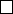5Fourier transform

II Probability and Measure5.1 The Fourier transform
When talking about Fourier transforms, we will mostly want to talk about
functions
R
d
C
. So from now on, we will write
L
p
for complex valued Borel
functions on R
d
with
kfk
p
=
Z
R
d
|f|
p
1/p
< .
The integrals of complex-valued function are defined on the real and imaginary
parts separately, and satisfy the properties we would expect them to. The details
are on the first example sheet.
Definition
(Fourier transform)
.
The Fourier transform
ˆ
f
:
R
d
C
of
f
L
1
(R
d
) is given by
ˆ
f(u) =
Z
R
d
f(x)e
i(u,x)
dx,
where u R
d
and (u, x) denotes the inner product, i.e.
(u, x) = u
1
x
1
+ ··· + u
d
x
d
.
Why do we care about Fourier transforms? Many computations are easier
with
ˆ
f
in place of
f
, especially computations that involve differentiation and
convolutions (which are relevant to sums of independent random variables). In
particular, we will use it to prove the central limit theorem.
More generally, we can define the Fourier transform of a measure:
Definition
(Fourier transform of measure)
.
The Fourier transform of a finite
measure µ on R
d
is the function ˆµ : R
d
C given by
ˆµ(u) =
Z
R
d
e
i(u,x)
µ(dx).
In the context of probability, we give these things a different name:
Definition
(Characteristic function)
.
Let
X
be a random variable. Then the
characteristic function of X is the Fourier transform of its law, i.e.
φ
X
(u) = E[e
i(u,X)
] = ˆµ
X
(u),
where µ
X
is the law of X.
We now make the following (trivial) observations:
Proposition.
k
ˆ
fk
kfk
1
, kˆµk
µ(R
d
).
Less trivially, we have the following result:
Proposition. The functions
ˆ
f, ˆµ are continuous.
Proof. If u
n
u, then
f(x)e
i(u
n
,x)
f(x)e
i(u,x)
.
Also, we know that
|f(x)e
i(u
n
,x)
| = |f(x)|.
So we can apply dominated convergence theorem with |f| as the bound.